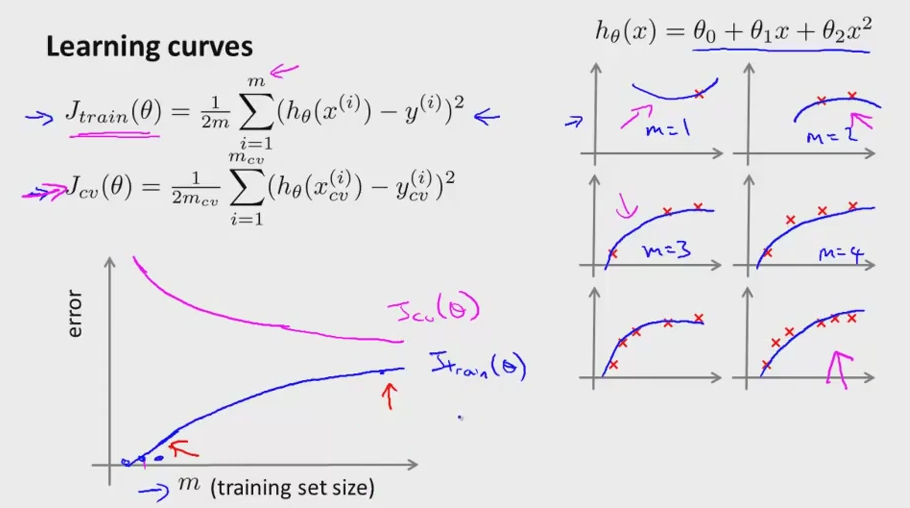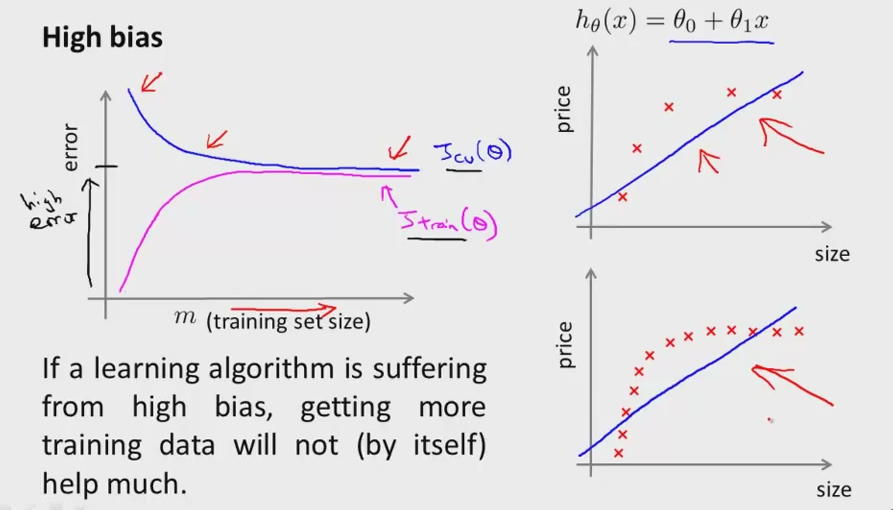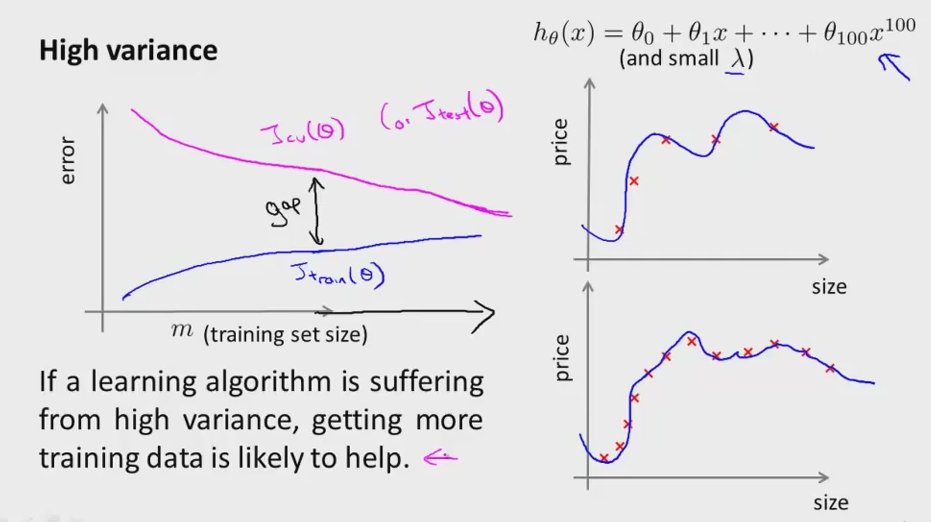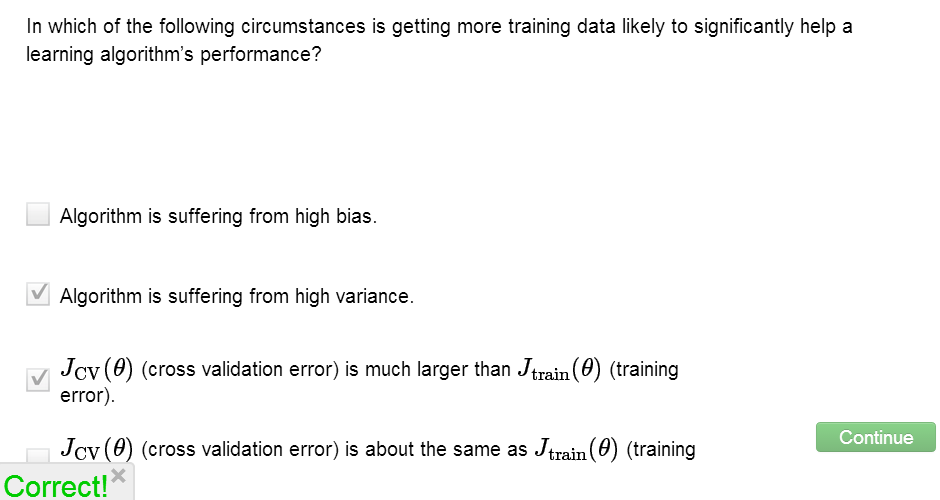• Curve to check the learning algorithm, whether bias, variance, or both• To plot learning curve, take small subset of training set(10-40 training examples)
• training set for m = 3, training set = 0. slightly larger if using regularization
• Learning curves will only make a shape only based on that three training example
• Say m >= 4, will become harder for the curve to shape all training set perfectly
• Concretely more training examples, the harder the curve to fit all the examples.
• The more data we have, the more fit the cross validation error, that is better hyphothesis for more training examples
• Sooo, with m start small, the error of Jtrain will be small, because it will overfit smaller data• This is what we get for the curve if what we have is either bias or variance
• First choosing the model (See hypothesis formula) that is only the linear model.
• The high bias can be shown as the three Jtest, Jtrain, and Jcv are all end up at high value
• suffering High Bias can't really help much if we get more training data.
• As we taught earlier, the Jcv will be similar to Jtrain if the learning suffer high bias, the error will be usually high.• Next is if we  suffering high variance, denotes by Jc) >>(much higher) Jtrain.
• Suppose we use hypothesis with a lot of high order polynomials and small lambda
• Jtrain if get more data, will generally close to future example,
• But more the data the cross validation will keep decreasing(less error), and the Jtrain will keep increasing(more error)
• Sometimes curve can be a lot cleaner/messier, but still helps out intuition if it has bias/variance problem• Next, discussed about spesific actions to take (or not take) to increase the performance of learning algorithm